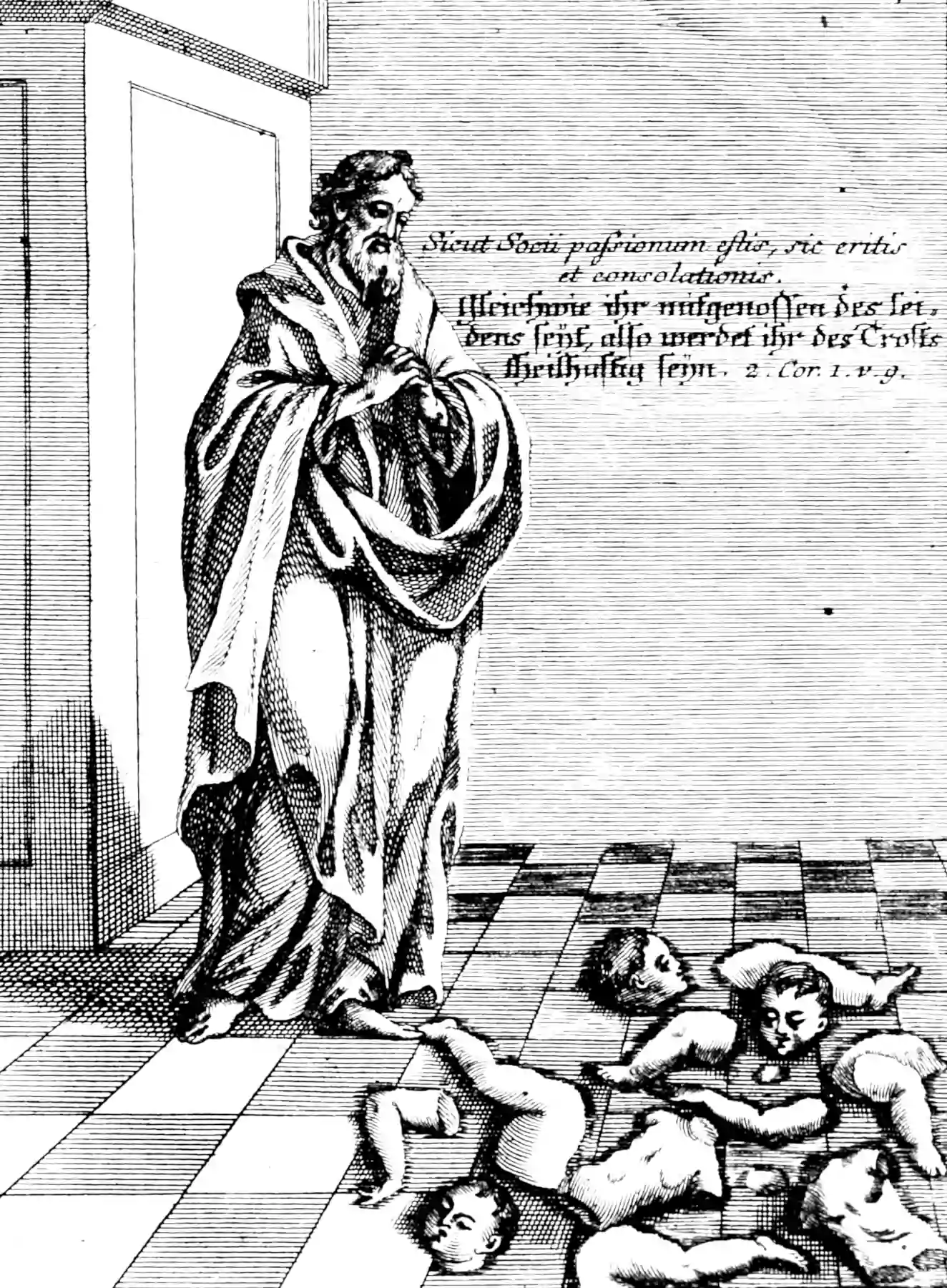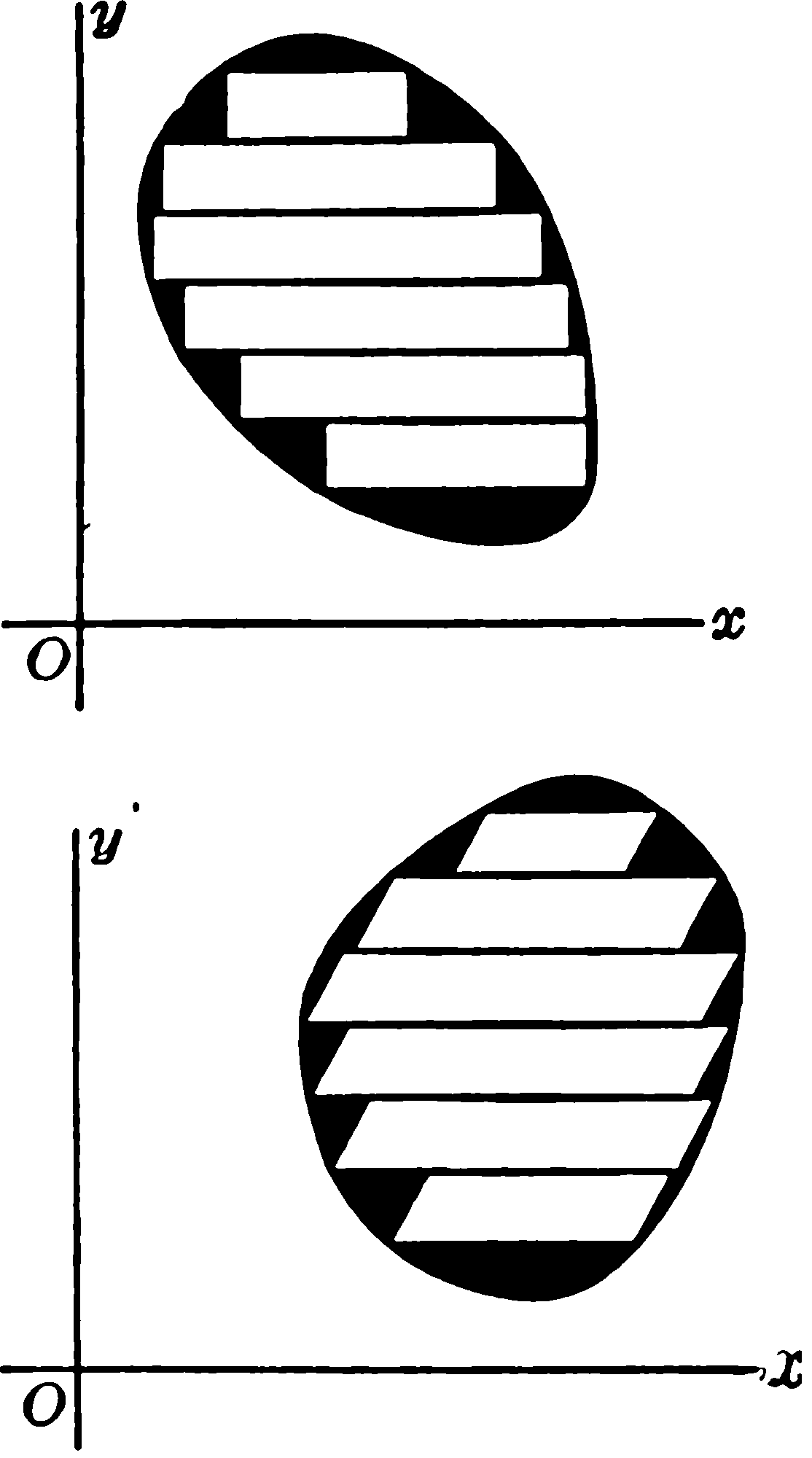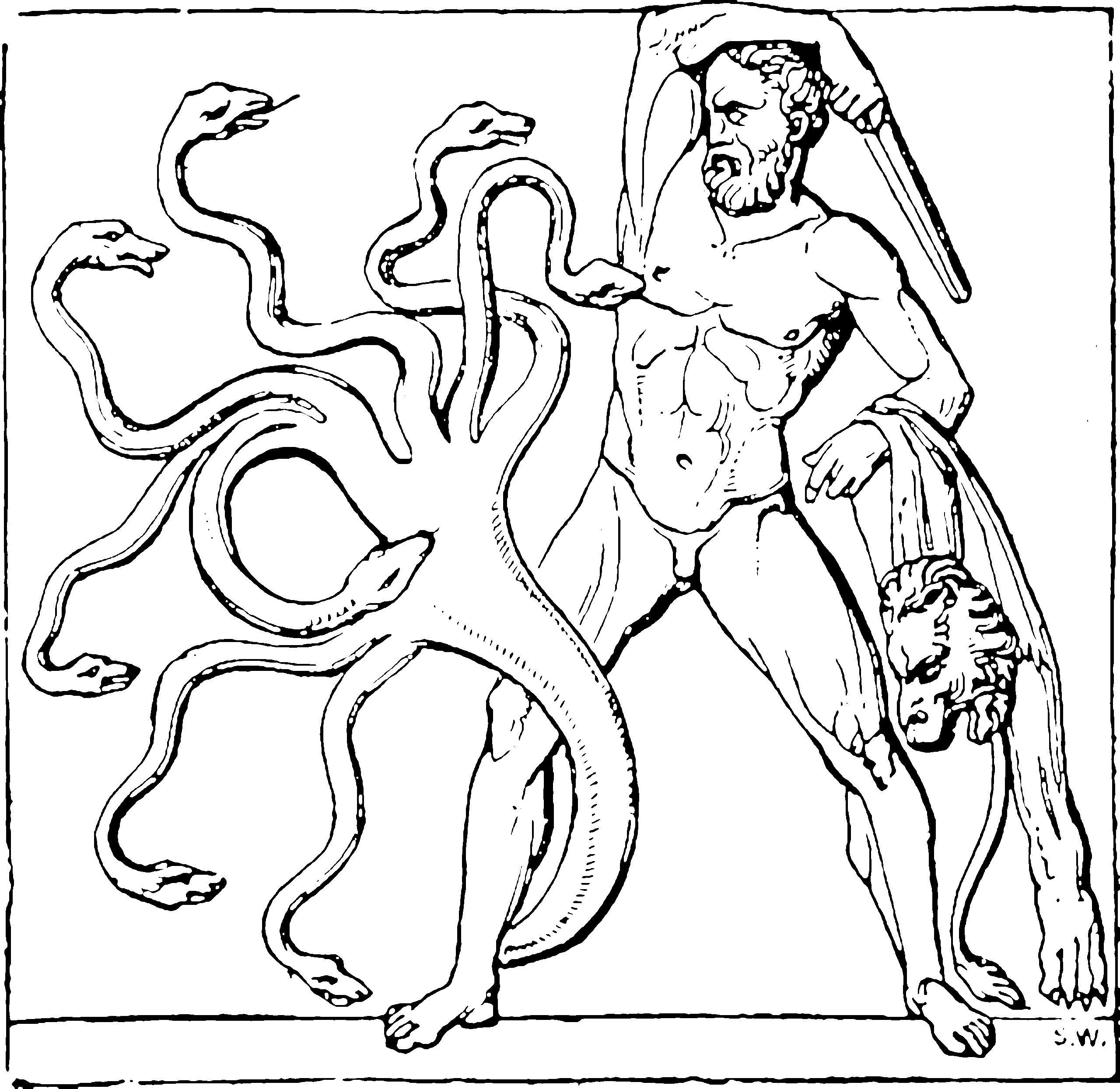# Factor graphsFor as the sufferings of dimensions abound in us, so our consolation aboundeth through localisation.

An alternative way of constructing graphical models which, loosely speaking, includes directed and undirected graphs by decomposing the model into more nodes and edges. I am currently interested in this because it seems to be pedagogically simpler than either of the other two DAG formalisms, of directed and undirected graphs, at least from some angles — we give up the on-to-one mapping between variables and nodes, but gain a much simpler algebra, especially in the Forney-style graphs.

Wikipedia tells us

A factor graph is a bipartite graph representing the factorization of a function. In probability theory and its applications, factor graphs are used to represent factorization of a probability distribution function, enabling efficient computations, such as the computation of marginal distributions through the sum-product algorithm.

That is, unlike the other two DAG formalisms, we we assign nodes to variables and a different type of node to relations between nodes.

To discuss: relation to message passing updates for variational inference and expectation propagation e.g. Cox and De Vries (2018) and Cox, van de Laar, and de Vries (2019).The insight of factor graphs is that we can turn this ⬆ problem into that ⬇ problem.We start from the Hammersley-Clifford Theorem and work backwards to the most convenient graph to represent it. Once the factor graph is written out (which is a slightly weird process) computations on the graph are essentially the same for every node. By contrast, in a classic I-graph DAG there are many different rules to remember for leaf nodes and branches, for colliders and forks etc.Loeliger’s 2004 zoo of factor graphs amongst other graphical models

## Classic factor graphs

TBD . This classic version is explained lots of places, but for various reasons I needed the FFG version first. I may return to classic factor graphs if needed.

## Forney-style factor graphs (FFGs)

An alternative formalism. Citations tell me this was introduce in a recondite article which I did not remotely understand because it was way too far into coding theory for me. It is explained and exploited much better for someone of my background in subsequent articles and used in several computational toolkits .

Relative to classic factor graphs, H.-A. Loeliger (2004) advocates for FFGs for the following advantages

• suited for hierarchical modeling ("boxes within boxes")
• compatible with standard block diagrams
• simplest formulation of the summary-product message update rule
• natural setting for Forney’s results on Fourier transforms and duality.

Mao and Kschischang (2005) argues:

Forney graphs possess a strikingly elegant duality property: by a local dualization operation, a Forney graph for a linear code may be transformed into another graph, called the dual Forney graph, which represents the dual code

The explanation in Cox, van de Laar, and de Vries (2019) gives the flavour of how graphs in this style work:Figure 1: In an FFG, edges correspond to variables and nodes represent factors that encode constraints among variables. A node connects to all edges that correspond to variables that occur in its factor function. For example, node $$f_{b}$$ connects to edges $$x_{1}$$ and $$x_{2}$$ since those variables occur in $$f_{b}\left(x_{1}, x_{2}\right)$$. Variables that occur in just one factor $$\left(x_{3}\right.$$ and $$x_{5}$$ in this case) are represented by half-edges. While an FFG is principally an undirected graph, we usually specify a direction for the (half-)edges to indicate the generative direction of the model and to anchor the direction of messages flowing on the graph.

A Forney-style factor graph (FFG) offers a graphical representation of a factorized probabilistic model. In an FFG, edges represent variables and nodes specify relations between variables. As a simple example, consider a generative model (joint probability distribution) over variables $$x_{1}, \ldots, x_{5}$$ that factors as $f\left(x_{1}, \ldots, x_{5}\right)=f_{a}\left(x_{1}\right) f_{b}\left(x_{1}, x_{2}\right) f_{c}\left(x_{2}, x_{3}, x_{4}\right) f_{d}\left(x_{4}, x_{5}\right),$ where $$f_{\bullet}(\cdot)$$ denotes a probability density function. This factorized model can be represented graphically as an FFG, as shown in Fig. 1. Note that although an FFG is principally an undirected graph, in the case of generative models we specify a direction for the edges to indicate the “generative direction”. The edge direction simply anchors the direction of messages flowing on the graph (we speak of forward and backward messages that flow with or against the edge direction, respectively). In other words, the edge directionality is purely a notational issue and has no computational consequences.…Figure 2: Visualization of the message passing schedule corresponding with observed variable $$x_{5}=\hat{x}_{5}$$. The observation is indicated by terminating edge $$x_{5}$$ by a small solid node that technically represents the factor $$\delta\left(x_{5}-\hat{x}_{5}\right) .$$ Messages are represented by numbered arrows, and the message sequence is chosen such that there are only backward dependencies. Dashed boxes mark the parts of the graph that are covered by the respective messages coming out of those boxes. The marginal posterior distribution $$f\left(x_{2} \mid x_{5}=\hat{x}_{5}\right)$$ is obtained by taking the product of the messages that flow on edge $$x_{2}$$ and normalizing.

The FFG representation of a probabilistic model helps to automate probabilistic inference tasks. As an example, consider we observe $$x_{5}=\hat{x}_{5}$$ and are interested in calculating the marginal posterior probability distribution of $$x_{2}$$ given this observation.

In the FFG context, observing the realization of a variable leads to the introduction of an extra factor in the model which “clamps” the variable to its observed value. In our example where $$x_{5}$$ is observed at value $$\hat{x}_{5}$$, we extend the generative model to $$f\left(x_{1}, \ldots, x_{5}\right) \cdot \delta\left(x_{5}-\hat{x}_{5}\right).$$ Following the notation introduced in Reller (2013), we denote such “clamping” factors in the FFG by solid black nodes. The FFG of the extended model is illustrated in Fig. 2

Computing the marginal posterior distribution of $$x_{2}$$ under the observation $$x_{5}=\hat{x}_{5}$$ involves integrating the extended model over all variables except $$x_{2},$$ and renormalizing: $f\left(x_{2} \mid x_{5}=\hat{x}_{5}\right) \propto \int \ldots \int f\left(x_{1}, \ldots, x_{5}\right) \cdot \delta\left(x_{5}-\hat{x}_{5}\right) \mathrm{d} x_{1} \mathrm{~d} x_{3} \mathrm{~d} x_{4} \mathrm{~d} x_{5}$ $=\overbrace{\int \underbrace{f_{a}\left(x_{1}\right)}_{1} f_{b}\left(x_{1}, x_{2}\right) \mathrm{d} x_{1}}^{2} \overbrace{\iint f_{c}\left(x_{2}, x_{3}, x_{4}\right) \underbrace{\left(\int f_{d}\left(x_{4}, x_{5}\right) \cdot \delta\left(x_{5}-\hat{x}_{5}\right) \mathrm{d} x_{5}\right)}_{3} \mathrm{~d} x_{3} \mathrm{~d} x_{4}}^{(4)} .$ The nested integrals result from substituting the [original] factorization and rearranging the integrals according to the distributive law. Rearranging large integrals of this type as a product of nested sub-integrals can be automated by exploiting the FFG representation of the corresponding model. The sub-integrals indicated by circled numbers correspond to integrals over parts of the model (indicated by dashed boxes in Fig. 2), and their solutions can be interpreted as messages flowing on the FFG. Therefore, this procedure is known as message passing (or summary propagation). The messages are ordered (“scheduled”) in such a way that there are only backward dependencies, i.e., each message can be calculated from preceding messages in the schedule. Crucially, these schedules can be generated automatically, for example by performing a depth-first search on the FFG.

What local means here is might not be intuitive. What we mean is that, in this high dimensional integral, only some dimensions are involved in each sub-step thanks to the multiplicative factorisation of the overall function.

Extension to systems without a density is left as an exercise for the student.

Question: These introductory texts discuss sum-product message passing, which is essentially about solving integrals. But what if I want to pass some other kind of update, e.g. expectation propagation? Then we no longer have exactly the same rational about factorising the form of the integral. Does this factor graph still help us?

There are some weird things about FFGs; For example, since a variable can only appear in two factors, if you want a variable to appear in more than 2, you add extra factors in, each of which constrains the variables touching it to be equal.

## Fourier transforms in

What does it benefit to take a fourier transform of a graph?

## In Bayesian brain models

See de Vries and Friston (2017) and van de Laar et al. (2018) for a connection to predictive coding.

## Causal inference in

How does do-calculus work in factor graphs?

### No comments yet. Why not leave one?

GitHub-flavored Markdown & a sane subset of HTML is supported.